## Looking for your content...Type it here

### GATE Previous Years Papers on Instrumentation Engineering

GATE 2010 PAPER-IN:INSTRUMENTATION ENGG

Q1-25 carry one mark each:

1) The infinite series f(x)= X3 /3! + X5 /5! + X7 /7!…………….
∞ converges to(a) cos(x)
(b)
sin(x)
(c)
sinh(x)
(d)
ex 2) The diameters of 10000 ball bearings were measured.The diameter and standard deviation were found to be 10mm and 0.05 mm respectively.Assuming gaussian distribution of measurements,it can be expected that the number of measurements more than 10.15 mm will be(a)230
(b)15
(c)115
(d)2

3)A person weighing 60 kg recieves radiation energy 0.3 j over the entire body.The dose of radiation absorbed(in rad) is

4) u(t) represents the unit step function.The laplace transform of u(t-τ) is

(a) 1/sτ
(b) 1/s-τ
(c)
e-sτ /s
(d)e-sτ

5) A measurement system with input x(t) and output y(t) is described by the differential eqation 3dy/dt +5y=8x.The stataic sensitivity of the system is
(a) 0.6
(b) 1.6
(c) 1.67
(d) 2.67

6) Poisson's ratio for a metal is 0.35.neglecting piezoresistance effect,the gauge factor of a strain gauge made of this metal is

(a) 0.65
(b) 1
(c) 1.35
(d) 1.70

7) Match the following :

 P. Radiation Pyrometer W. Angular velocity measurement Q. Dall tube X. Vacuum pressure measurement R. Pirani gauge Y. Flow measurement S. Gyroscope Z. Temperature measurement
(a) P-Z,Q-W,R-X,S-Y
(b) P-Z,Q-Y,R-X,S-W
(c) P-W,Q-X,R-Y,S-Z
(d) P-Z,Q-X,R-W,S-Y
8) In a pulse code modulated (PCM) signal sampled at fs and encoded into an n-bit code,the minimum bandwidth required for faithful reconstruction is(a) 2nfs
(b) n
fs

(c) nf
s
(d)
fs

9) A beam of unpolarized light is first passed through a linear polarizer and then through a quarter wave plate.The emergent beam is

(a) unpolarized
(b) linearly polarized
(c) circularly polaized
(d) elliptically polaized

10) f(x),shown in the adjoining figure is represented by

f(x)=
a +(a) 0
(b) π/2
(c)
π
(d) 2
π

11) The PMMC ammeter A in the adjoining figure has a range of 0 to 3 mA.when switch S1 is opened,the pointer of the ammete swings to the 1 mA mark,returns and settles at 0.9 mA. The meter is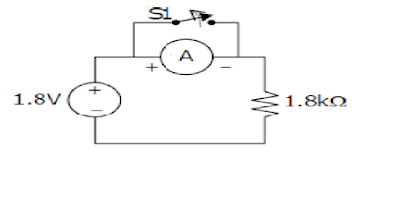(a) critically damped and has a coil resistance of 100
Ω
(b) critically damped and has a coil resistance of 200
Ω
(c) under damped and has a coil resistance of 100
Ω
(d) under damped and has a coil resistance of 200
Ω

12) The open loop transfer function of a unity gain feedback system is given by:

G(s) =k(s+3)/(s+1)(s+2)

The range of positive values of k for which the closed loop system will remain stable is:

(a)

13) AREAL MATRIX A=[a] is defined as follows:

a=i, if i=j
=0,otherwise
The summation of all n eigenvalues of A is

(a) n(n+1)/2
(b) n(n-1)/2
(c) n(n+1)(2n+1)/2
(d)
n2

14) The contour C in the adjoining figure is described by
x­­­2 +y­2 =16The value of
∮z­­­­­­2 +8/0.5z-1.5j dz is
(Note: j=
√1 )

(a) -2πj
(b) 2πj

(c)4
πj
(d)-4πj

15) In the dc circuit shown in the adjoining figure, the node voltage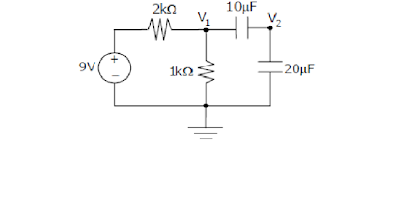(a) 0 v (b) 1 v (c) 2 v (d) 3 v

16) A 100 Ω, 1 w resistor and a 800 Ω, 2 w resistor are connected in series.The maximum dc voltage that can beapplied continously to the series circuit without exceeding the power limit of any of the resistors is

(a) 90 v
(b) 50 v
(c) 45 v
(d) 40 v

17) The seismic mass of an accelerometer oscillates sinusoidally at 100 hz with a maximum displacement of 10 mmfrom its mean position.The peak acceleration of the seismic mass is

(a) 3947.84 m/
s­2
(b) 3141.50
m/s­2
(c) 314.15
m/s­2
(d) 100.00
m/s­2

18) In the ideal op amp circuit given in the adjoining figure,the value of is varied from 1 k
Ω to 100 kΩ. The gain G = will(a) remain constant at +1
(b) remain constant at -1
(c) vary as -()
(d) vary as()

19) A signal with frequency components 50 hz,100 hz and 200 hz only is sampled at 150 samples/s.The ideally reconstructed signal will have frequency component(s) of

(a) 50 hz only (b) 75 hz only (c) 50 hz and 75 hz (d) 50 hz,75 hz and 100 hz.

20) The subroutine SBX given below is executed by an 8085 processor.The value in the accumulator immediately after the execution of the subroutine will be:

SBX: MVI A,99h
MOV C,A
RET
(a) 00h (b) 11h (c) 99h (d) AAh

21) The integral evaluates to

(a) 6 (b) 3 (c) 1.5 (d) 0

22) The deflection angle of the pointer of an ideal moving iron ammeter is for 1.0 ampere dc current.If a current of 3sin(314t) amperes is passed through the ammeter then the deflection angle is

(a)

23) AN 8 bit DAC is interfaced witha microprocessor having 16 address lines () as shown in the adjoining figure.A possible valid address for this DAC is(a) 3000h
(b) 4FFFh
(c) AFFFh
(d) C000h

24) H(z) is a discrete rational transfer function .To ensure that both H(z) and its inverse are stable its

(a) poles must be inside the unit circle and zeros must be outside the unit circle.
(b) poles and zeros must be inside the unit circle.
(c) poles and zeros must be outside the unit circle.
(d) poles must be outside the unit circle and the zeros should be inside the unit circle.

25) The output voltage of a transducer with an output resistance of 10 k
Ω is connected to an amplifier.The minimum input resistance of the amplifier so that the error in recording the transducer output does not exceed 2% is

(a) 10 k
Ω (b) 49 kΩ (c) 490 kΩ (d) 1.2MΩQ26-55 carry two mark each:

26) X and Y are non zero square matrices of size n x n. if XY= then

(a)|X| = 0 and |Y| ≠ 0
(b)|X| ≠ 0 and |Y| = 0
(c)|X| = 0 and |Y| = 0
(d)|X| ≠ 0 and |Y| ≠ 0

27) Consider the differential equation dy/dx + y = with y(0)=1. The value of y(1) is
(a)

28) The electric charge density inthe region is given as where x and y are in meters.The total charge (in columb) contained in the region R is

(a) 4π (b) 2π (c) π/2 (d) 0
29) The input x(t) and the corresponding output y(t) of a system are related by y(t) =
the system is
(a) time invarient and causal
(b) time invarient and non causal
(c) time varient and non causal
(d) time varient and causal
30) A digital filter having a transfer function H(z) = is implemented using Direct form- I and direct form II realizations are, respectively
(a) 6 and 6 (b) 6 and 3 (c) 3 and 3 (d) 3 and 2
31) The velocity v(in m/s) of a moving mass, starting from rest, is given as dv/dt=v+t.Using Euler forward difference method(also known as Cauchy- Euler method) with a step size of 0.1 s,the velocity at 0.2 sec evaluates to
(a) 0.01 m/s (b) 0.1 m/s (c) 0.2 m/s (d) 1 m/s
32) The rotor of the control transformer of a synchro pair gives a maximum voltage of 1.0 v at a particular position of the rotor of the control transmitter.The transmitter rotor is now rotated by 30 anticlockwise keeping the transformer rotor stationary.The transformer rotor voltage for this position is
(A) 1.0v (b) 0.866v (c) 0.5v (d) 0v
33) The matched transistors Q1 and Q2 shown in the adjoining figure have =100.Assuming the base emitter voltages to be 0.7v.The collector emitter voltage of the transistor Q2 is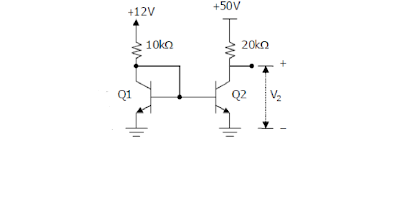(a) 33.9 v
(b) 27.8 v
(c) 0.5 v
(d) 0 v

34) The volume of a cylinder is computed from measurements of its height (h) and diameter(d). A set of several measurements of height has an average value of 0.2 m and a standard of 1%.The average value obtained for the diameter is 0.1 m and the standard deviation is 1%assuming the errors in the measurements of height and diameter are uncorrelated,the standard deviation of computed volume is
(a) 1.00% (b) 1.73% (c) 2.23% (d) 2.41%
35) A thermocouple based temperature system is shown in the adjoining figure.Relevant thermocouple emf data (in mV) is given below.The cold junction is kept at .The temperature is in the other parts of the system.The emf is measured to be 26.74 mV. The temperature of the hot liquid is

 Temperature Emf of Chromel- Constantan Emf of Copper- Constantan 10 ºC 0.591 0.391 20 ºC 1.192 0.789 30 ºC 1.801 1.196 370 ºC 26.549 9.027 380 ºC 27.345 19.638

(a) 370.0 (b) 372.4 (c) 376.6 (d) 380.0
36)A differntial pressure transmitter is used to measure the flow rate in a pipe.Due to aging the sensitivity of the pressure transmitter is reduced by 5%.All other aspects of the flow meter remaining constant,change in the sensitivity of the flow measurement is
(a) 10.0% (b) 5.0% (c) 2.5 % (d) 2.2%
37) The asymptotic bode magnitude plot of a lead network with its pole and zero on the left half of the s-plane is shown in the adjoining fig. The frequency at which the phse angle of the network is maximum(in rad/s) is

(a) 3 (b) 1 (c) 1/20 (d) 1/30

38)In an analog single channel cathode ray oscillioscope (cro),the x and y sensitivities are set as 1 ms/div and 1 v/div. respectively.The y input is connected to a voltage signal 4 cos v..The trigger source is internal,level chosen is zero and the slope is positve.The display seen on the cro screen is

39) A unit ramp input is applied to the system shown in the adjoining figure.The steady state error in its output is

(a) 0 (b) 0.5 (c) 1 (d) 2

40) A unity feedback system has an open loop transfer function G(s) = k/s(s+3) .The value of k that yields a damping ratio of o.5 for the closed loop system is
(a) 1 (b) 3 (c) 5 (d) 9
41) A 4 bit successive approximation type ADC has a full scale value of 15 v.The sequence of the states,the SAR will traverse,for the conversion of an input of 8.15 v is

42) The logic gate circuit shown in the adjoining figure realizes the function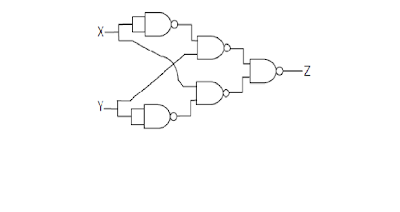43)In an 8085 processor, the main program calls the subroutine SUB1 given below. When the program returns to the main program after executing SUB1, the value in the accumulator is

2000 3E 00
2002 CD 05 20
2005 3C
2006 C9

SUB1: MVI A,00h
CALL SUB2
SUB2: INR A
RET

(a) 00 (b) 01 (c) 02 (d) 03

44) Light coming out of an optical fiber is incident on a plane perpendicular to the fiber axis and 50mm away from the end of the fiber. The light coming out creates a circular spot that can at most be of 20mm diameter. Neglecting the diameter of the fiber, the numerical aperture of the fiber is,approximately
(A) 0.14
(B) 0.20
(C) 0.34
(D) 0.40

45) A solution “P” is put in a spectrophotometer cuvette of optical path length 1cm. The transmittance is found to be 10%. Another solution “Q” has a transmittance of 40% under the same circumstances. If equal volumes of P and Q are mixed together, the transmittance of the resulting solution (assuming the constituents of P and Q do not react with each other) is, approximately,

(A) 15%
(B) 20%
(C) 25%
(D) 30%

46) 4-point DFT of a real discrete-time signal x[n] of length 4 is given by X[k ], n= 0,1 2, 3, and k =0,
1, 2 ,3.It is given that X =5,X=1+ j1, X=0.5 . X and X respectively are
(A) 1-j, 1.875
(B) 1-j, 1.500
(C) 1+j, 1.875
(D) 0.1-j0.1, 1.500

47) An active filter is shown in the adjoining figure. The dc gain and the 3dB cut-off frequency of the
filter respectively, are, nearlyR1 = 15.9 k Ω , R2 = 159 k Ω , C1 = 1.0nF
(A) 40dB, 3.14 kHz
(B) 40dB, 1.00 kHz
(C) 20dB, 6.28 kHz
(D) 20dB, 1.00 kHz

Common Data for Questions: 48 & 49
A differential amplifier is constructed using an ideal opamp as shown in the adjoining figure. The values of R1 and R2 are 47k Ω and 470k Ω respectively.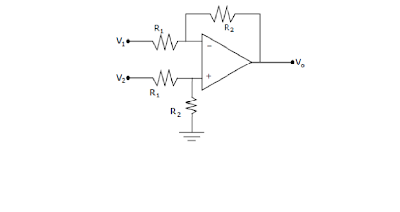48. The input impedances seen looking into the terminals V1 and V2, with respect to ground,
respectively are

(A) 47k Ω and 43k Ω
(B) 47k Ω and 47k Ω
(C) 47k Ω and 517k Ω
(D) 517k Ω and 517k Ω

49) V1 and V2 are connected to voltage sources having an open circuit output of +1V each and internal resistances of 13k Ω and 3k Ω respectively. The output voltage V0 is
(A) 0V
(B) 0.15V
(C) 1.5V
(D) 10V

Common Data for Questions: 50 & 51

A PMMC type ammeter has full scale current of 100 μA and a coil resistance of
100 Ω

50. The resistance required to convert the 100 μA ammeter into 1A full scale dc ammeter is

(A) 10mΩ in series with the meter
(B) 10mΩ in parallel with the meter
(C) 1mΩ in series with the meter

51) The above PMMC meter is connected in the circuit shown in the adjoining figure. The opamp is ideal. The voltage vi(t) = 1.0sin314t V . Assuming the source impedance of vi (t) to be zero, the ammeter will indicate a current of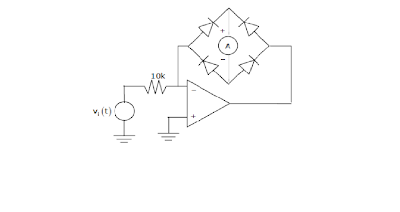(A) 100μA
(B) 70.7 μA
(C) 63.7 μA
(D) 31.8 μA

A coil having an inductance (L) of 10mH and resistance R is connected in series with an ideal 100 μ F capacitor (C). When excited by a voltage source of value 10√2 (1000t)V, the series RLC circuit draws
20W of power.

52) The value of the coil resistance R is
(A) 1 Ω
(B) 2 Ω
(C) 4 Ω
(D) 5 Ω

53) The Q factor of the coil at an angular frequency of 1000rad/s is
(A) 1
(B) 2
(C) 4
(D) 5

Consider a temperature measurement scheme shown in the adjoining figure. It uses an RTD whose resistance at 0ºC is 100Ω and temperature coefficient of resistance ( α ) is 0.00392/ºC.

54)The differential gain of the instrumentation amplifier to achieve a voltage sensitivity of 10mV/ºC at 0ºC should be approximately
(A) 13.41
(B) 26.02
(C) 57.53
(D) 90.14

55) The RTD is placed in a hot water bath of temperature 100ºC. Based on the gain calculated in Q.54, the error in the measured value of the temperature due to bridge nonlinearity is
(A) -0.1ºC
(B) -0.4 ºC
(C) -0.9 ºC
(D) +1.2 ºC

General Aptitude (GA) Question

Q. 56 – Q. 60 Carry One Mark Each.

56. 25 persons are in a room. 15 of them play hockey, 17 of them play football and 10 of them play both hockey and football. Then the number of persons playing neither hockey nor football is:
(A) 2
(B) 17
(C) 13
(D) 3

57) Choose the most appropriate word from the options given below to complete the following sentence: If we manage to _______________________ our natural resources, we would leave a better planet for our
children.
(A) uphold
(B) restrain
(C) cherish
(D) conserve

58) The question below consists of a pair of related words followed by four pairs of words. Select the pair that best expresses the relation in the original pair.
Unemployed : Worker
(A) fallow : land
(B) unaware : sleeper
(C) wit : jester
(D) renovated : house

59) Which of the following options is the closest in meaning to the word below:
Circuitous
(A) cyclic
(B) indirect
(C) confusing
(D) crooked

60) Choose the most appropriate word from the options given below to complete the following sentence:His rather casual remarks on politics _____________________ his lack of seriousness about the
subject.
(B) belied
(C) betrayed
(D) suppressed

Q. 61 – Q. 65 Carry Two Marks Each.

61) Hari (H), Gita (G), Irfan (I) and Saira (S) are siblings (i.e. brothers and sisters). All were born on 1st January. The age difference between any two successive siblings (that is born one after another) is less than 3 years. Given the following facts:
i. Hari’s age + Gita’s age > Irfan’s age + Saira’s age
ii. The age difference between Gita and Saira is 1 year. However, Gita is not the oldest and Saira is not the youngest.
iii. There are no twins.

In what order were they born (oldest first)?

(A) HSIG
(B) SGHI
(C) IGSH
(D) IHSG

62) 5 skilled workers can build a wall in 20 days; 8 semi – skilled workers can build a wall in 25 days; 10 unskilled workers can build a wall in 30 days. If team has 2 skilled, 6 semi-skilled and 5 unskilled workers,how long will it take to build the wall?

(A) 20 days
(B) 18 days
(C) 16 days
(D) 15 days

63) Modern warfare has changed from large scale clashes of armies to suppression of civilian populations.Chemical agents that do their work silently appear to be suited to such warfare; and regretfully, there exist people in military establishments who think that chemical agents are useful tools for their cause.

Which of the following statements best sums up the meaning of the above passage;

(A) Modern warfare has resulted in civil strife.
(B) Chemical agents are useful in modern warfare.
(C) Use of chemical agents in warfare would be undesirable.
(D) People in military establishments like to use chemical agents in war.

64) Given digits 2, 2, 3, 3,3, 4, 4, 4, 4 how many distinct 4 digit numbers greater than 3000 can be formed?

(A) 50
(B) 51
(C) 52
(D) 54

65) If 137 +276 = 435 how must is 731 + 672?

(A) 534
(B) 1403
(C) 1623
(D) 151